Sie sind hier: ICP » R. Hilfer » Publikationen

# 4 Inverse Mittag-Leffler functions

[647.7.1] We introduce the inverse generalized Mittag-Leffler functions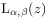as the solutions of the equation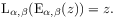(43)

[647.7.2] Our ability to calculate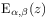allows us to evaluate alsoby solving this functional equation numerically. [647.7.3] We have succeeded to determine the principal branch ofin such a way that three conditions are fulfilled. [647.7.4] 1. The functionis single valued and well defined on its principal branch. [647.7.5] 2. Its principal branch reduces to the principal branch of the logarithm for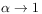. [647.7.6] 3. Its principal branch is a simply connected subset of the complex plane. [647.7.7] Figure 15 shows the principal branch for the case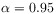,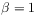.Figure 15: The dark region corresponds to the principal branch of the inverse generalized Mittag-Leffler functionfor,.